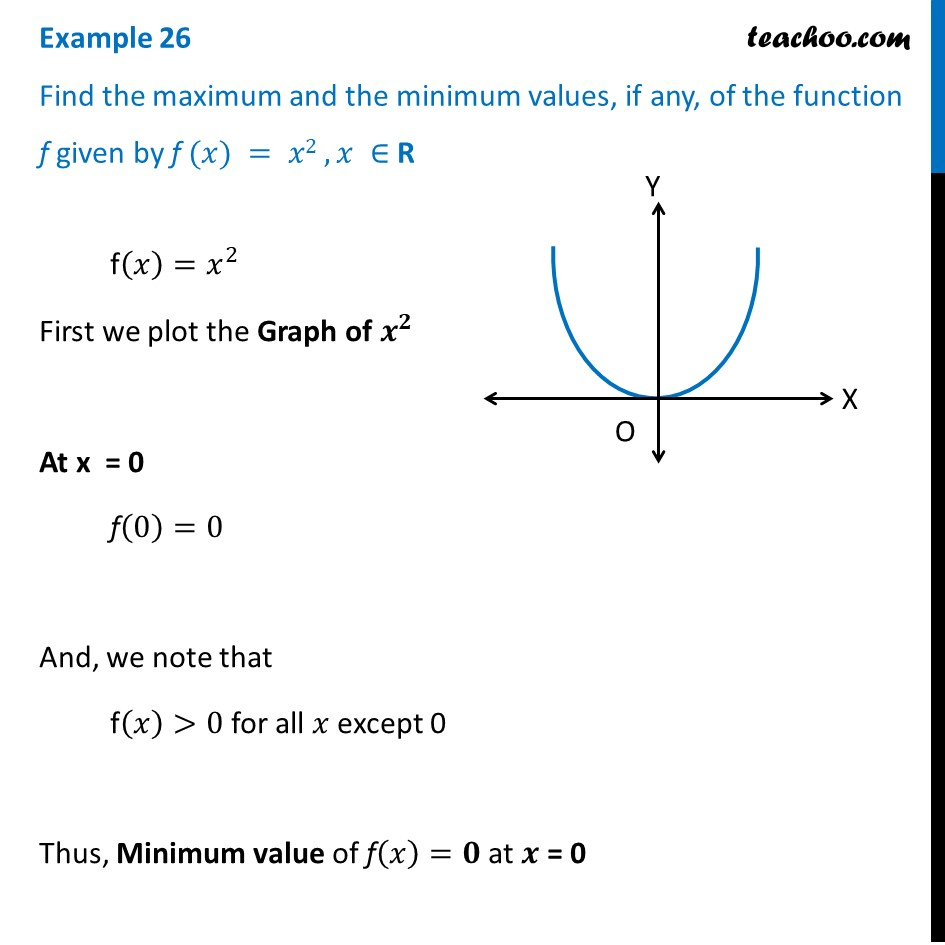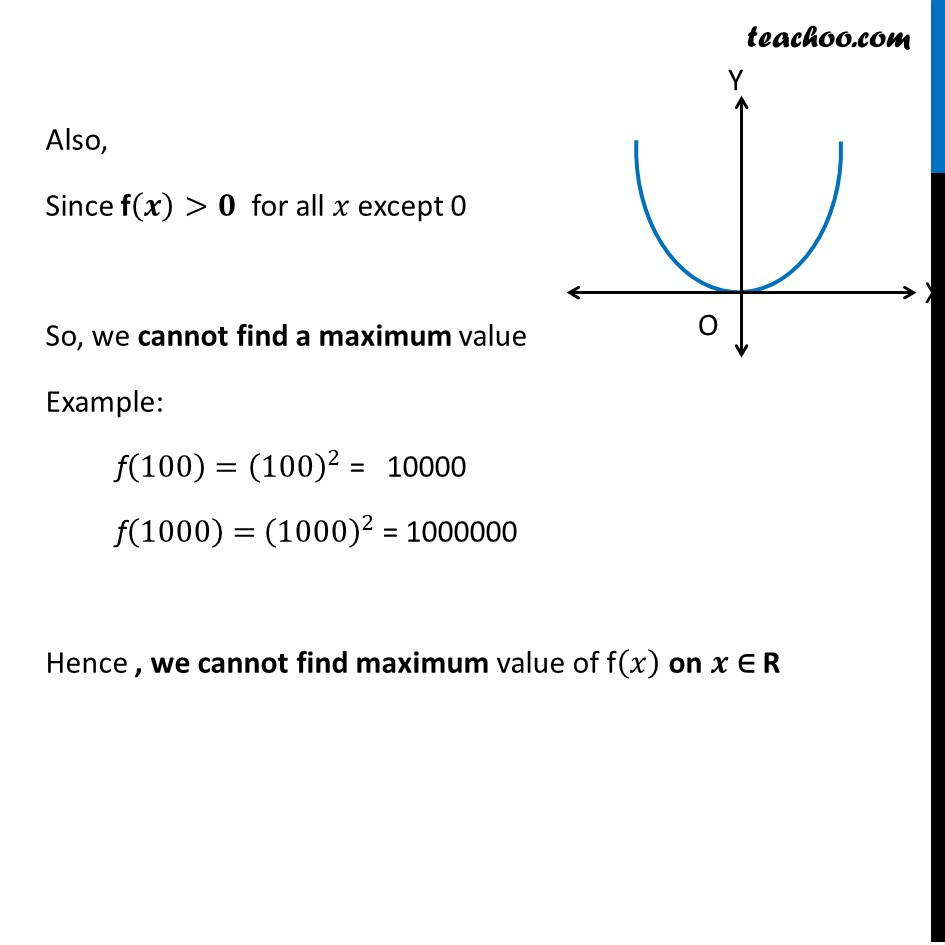Examples

Chapter 6 Class 12 Application of Derivatives
Serial order wiseLearn in your speed, with individual attention - Teachoo Maths 1-on-1 Class

### Transcript

Example 14 Find the maximum and the minimum values, if any, of the function f given by f (𝑥) = 𝑥2 , 𝑥 ∈ R f(𝑥)=𝑥^2 First we plot the Graph of 𝒙^𝟐 At x = 0 f(0)=0 And, we note that f(𝑥)>0 for all 𝑥 except 0 Thus, Minimum value of f(𝑥)=𝟎 at 𝒙 = 0 Also, Since f(𝒙)>𝟎 for all 𝑥 except 0 So, we cannot find a maximum value Example: f(100)=(100)^2 = 10000 f(1000)= (1000)^2 = 1000000 Hence , we cannot find maximum value of f(𝑥) on 𝒙 ∈ R